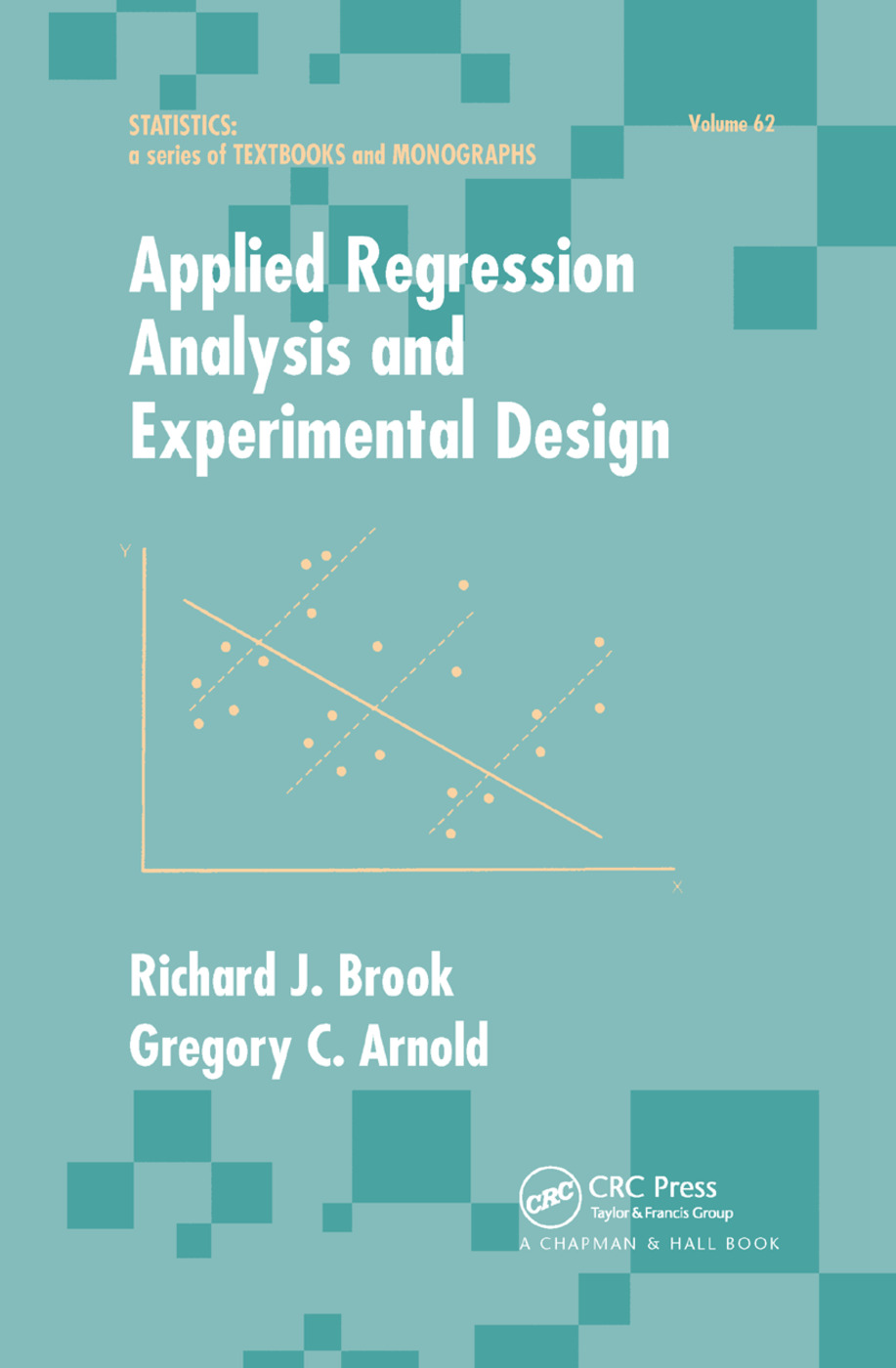# Applied Regression Analysis and Experimental Design

## 1st Edition

CRC Press

256 pages

Paperback: 9780367403416
pub: 2019-09-27
\$74.95
x
Hardback: 9780824772529
pub: 1985-04-25
\$150.00
x
eBook (VitalSource) : 9781315137674
pub: 2018-12-13
from \$28.98

FREE Standard Shipping!

### Description

For a solid foundation of important statistical methods, the concise, single-source text unites linear regression with analysis of experiments and provides students with the practical understanding needed to apply theory in real data analysis problems.

Stressing principles while keeping computational and theoretical details at a manageable level, Applied Regression Analysis and Experimental Design features an emphasison vector geometry and least squares to unify and provide an intuitive basis for most topics covered… abundant examples and exercises using real-life data sets clearly illustrating practical of data analysis…essential exposure to MINITAB and GENSTAT computer packages , including computer printouts…and important background material such as vector and matrix properties and the distributional properties of quadratic forms.

Designed to make theory work for students, this clearly written, easy-to-understand work serves as the ideal texts for courses Regression, Experimental Design, and Linear Models in a broad range of disciplines. Moreover, applied statisticians will find the book a useful reference for the general application of the linear model.

Preface Fitting a Model to Data Introduction How to Fit a Line Residuals Transformations to Obtain Linearity Fitting a Model Using Vectors and Matrices Deviations from Means An Example- Value of a Postage Stamp Over Time Problems Goodness of Fit of the Model Introduction Coefficient Estimates for Univariate Regression Coefficient Estimates for Mulitvariate Regression ANOVA Tables The F Test The Coefficient of Determination Predicted Values of Y and Confidence Intervals Residuals Reduced Models Pure Error and Lack of Fit Example- Lactation Curve Problems Which Variable Should Be Included in the Model Introduction Orthogonal Predictor Variables Linear Transformations of the Predictor Variables Adding Nonorthogonal Variables Sequentially Correlation Form Variable Selection- All Possible Regressions Variable Selection- Sequential Methods Qualitative (Dummy) Variables Aggregation of Data Problems Peculiarities of Observations Introduction Sensitive or High Leverage Points Outliers Weighted Least Squares More on Transformations Eigenvalues and Principal Components Ridge Regression Prior Information Cleaning up Data Problems The Experimental Design Model Introduction What Makes an Experiment The Linear Model Tests of Hypothesis Testing of Assumptions Problems Assessing the Treatment Methods Introduction Specific Hypothesis Contrasts Factorial Analysis Unpredicted Effects Conclusion Problems Blocking Introduction Structure of Experimental Units Balanced Incomplete Block Designs Confounding Miscellaneous Tricks Problems Extensions to the Model Introduction Hierarchic Designs Repeated Measures Covariance Analysis Unequal Replication Modeling the Data Problems Appendix A Review of Vectors and Matrices Some Properties of Vectors Some Properties of Vector Spaces Some Properties of Matrices Appendix B Expectation, Linear and Quadratic Forms Expectations Linear Forms Quadratic Forms The F-Statistic Appendix C Data Sets Ultra-Sound Measurements of Horses Hearts Ph Measurement of Leaf Protein Lactation Records of Cows Sports Cars House-Price Data Computer Teaching Data Weedicide Data References Index